Checkout JEE MAINS 2022 Question Paper Analysis : Checkout JEE MAINS 2022 Question Paper Analysis :

# Dipole Moment Questions

A bond dipole moment quantifies the polarity of a chemical bond formed by two atoms in a molecule. It makes use of the concept of the electric dipole moment, which is a measure of the separation of negative and positive charges in a system.

Since it has both magnitude and direction, the bond dipole moment is a vector quantity.

 Definition: A dipole moment occurs in any system where there is charge separation. As a result, they can form in both ionic and covalent bonds. Dipole moments occur as a result of an electronegativity difference between two chemically bonded atoms.

## Dipole Moment Chemistry Questions with Solutions

Q1. Which molecule has the largest dipole moment?

a) HCl

b) HI

c) HBr

d) HF

Explanation – Since the electronegativity difference between H and F is large, it has the largest dipole moment and is highly polar.

Q2. Which of the following molecules will show dipole moment?

a) Methane

b) Carbon tetrachloride

c) Chloroform

d) Carbon dioxide

Explanation – Chloroform is polarised and has a dipole moment because it has three chlorine atoms and one hydrogen atom attached to the carbon.

Q3. Which of the following is not correct for the dipole moment?

a) lone pair of electrons present on the central atom can give rise to the dipole moment

b) the dipole moment is a vector quantity

c) CO2 molecule has no dipole moment since C – O bonds are nonpolar

d) the difference in electronegativities of combining atoms can lead to the dipole moment

Correct Answer: (c) CO2 molecule has no dipole moment since C – O bonds are nonpolar.

Q4. Which of the following has greater observed dipole moment than theoretical dipole moment?

a)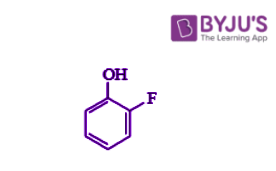b)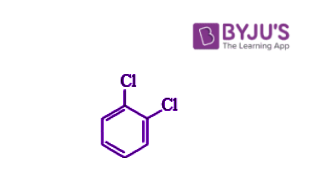c)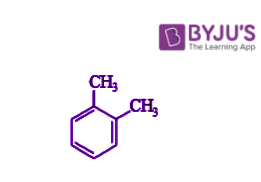d)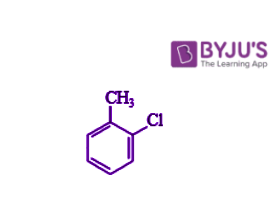Correct Answer: a)Q5. A diatomic molecule has a dipole moment of 1.2D. If its bond distance is 1.0Å, what fraction of electric charge “e” exists on each atom?

a) 12% of e

b) 18% of e

c) 25% of e

d) 30% of e

Correct Answer: c) 25% of e

Q6. Define dipole moment.

Answer. The dipole moment is defined as the product of the charge magnitude and the distance between the positive and negative charge centres.

Q7. Is the dipole moment a scalar or a vector quantity?

Answer. A dipole moment is a vector quantity represented by a small arrow with a tail pointing towards the positive centre and a head pointing towards the negative centre.

Q8. Why is the dipole moment of CO2, BF3, and CCl4 zero?

Answer. Since the molecules are symmetrical, the dipoles cancel out and the net dipole moment is zero.

Q9. Give the mathematical expression of dipole moment.

Answer. The dipole moment (M) is expressed mathematically as dipole moment (M) = charge (Q) x distance of separation (r). Debye units are commonly used to express dipole moment (D).

Q10. BeF2 has a dipole moment of zero. Give an explanation.

Answer. The dipole moment in BeF2 is zero because the two equal bond dipoles point in opposite directions and cancel each other out.

Q11. State some applications of Dipole moment?

Answer. It is used to compute the percentage ionic character, bond angle, electric polarisation, and residual charge of atoms in molecules. It also aids in determining the size or shape of molecules, as well as the arrangement of chemical bonds within them.

Q12. What is the % ionic character of HBr when the dipole moment of HBr is 1.6 × 10–30 cm and interatomic spacing is 1Å.

Answer. Charge of electron (q) = 1.6 × 10–19 C

Dipole moment of HBr = 1.6 × 10–30 cm

Inter- atomic spacing = 1Å = 1 × 10–10 m

% of ionic character in HBr = (dipole moment of HBr × 100) / (inner spacing distance × q)

% of ionic character in HBr = (1.6 × 10–30 × 100) / (1.6 × 10–19 × 10–10)

% of ionic character in HBr = 10 %

Q13. What is the dipole moment of CH4. Why?

Answer. Methane CH4 has a dipole moment of zero.

The CH4 molecule is tetrahedral in shape, and because of this, each bond pair is at equal distance, indicating that they are symmetrically arranged, and thus each dipole moment of the bond balances each other.

Q14. Is SO2 a polar molecule?

Answer. Since, CO2 is linear, its dipoles cancel. However, because SO22 is bent, the dipoles do not cancel, resulting in a polar molecule.

Q15. Which of the following would be expected to have a dipole moment of zero on the basis of symmetry? Explain.

a. SOCl2.

b. SiF4.

c. OF2.

a.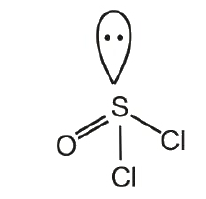Since there are two chlorine atoms on one side and two oxygen atoms on the other, this molecule is asymmetrical. There is also a solitary pair of electrons. Because it is asymmetrical, the charge distribution is uneven. As a result, it has a dipole moment that is greater than zero.

b.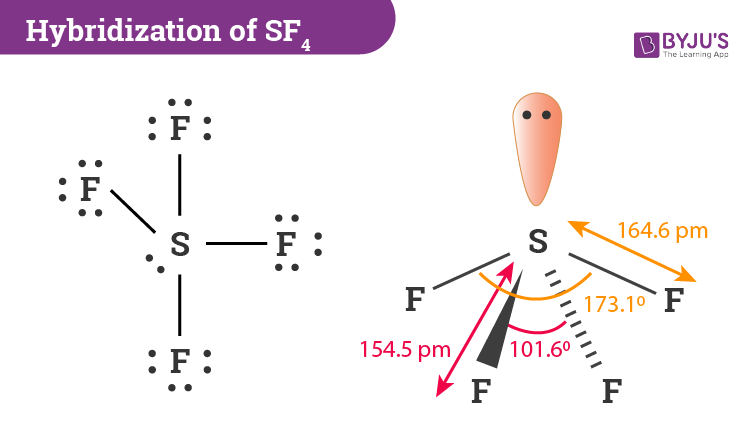Fluorine has a higher electronegativity than silicon. As a result, the dipole moment will be from the silicon atom to the fluorine atom. Above silicon is one fluorine atom. As a result, the dipole moment will be cancelled. Furthermore, because silicon is bonded to four fluorine atoms, this molecule is completely symmetrical.

So, SiF4 has zero dipole moment.

c.The electronegativity of oxygen is lower than that of fluorine. As a result, the dipole moment will be from oxygen to fluorine. Furthermore, this molecule has a twisted shape. As a result, instead of cancelling the charges, they are added.

So, OF2 has non-zero dipole moment.

## Practise Questions on Dipole Moment

Q1. The dipole momentum is directed ____.

a) From positive to negative charge

b) From negative to positive charge

c) From the center to the negative charge f the dipole only

d) From the positive charge to center of the dipole only

Q2. When an electric dipole is placed in the uniform electric field, ____ will be effective on it.

a) Force

b) Force and Torque

c) Torque

d) None of these

Q3. If HCl molecule is completely polarized, so expected value of dipole moment is 6.12D (deby), but experimental value of dipole moment is 1.03D. Calculate the percentage ionic character

a) 17

b) 83

c) 50

d) Zero

Q4. In the case of polyatomic molecules, what factors influence the dipole moment?

Q5. Why does NH3 have a higher dipole moment than NF3 despite the fact that both are pyramidal?

Click the PDF to check the answers for Practice Questions.

Click the PDF to check the answers for Practice Questions.

## Recommended Videos

#### Dipole Moment#### Electric Field of a Dipole